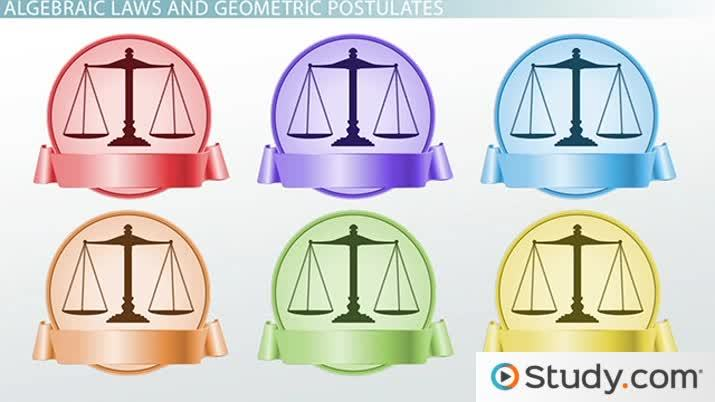# Algebraic Laws and Geometric Postulates

Lesson Transcript
Instructor: Yuanxin (Amy) Yang Alcocer

Amy has a master's degree in secondary education and has been teaching math for over 9 years. Amy has worked with students at all levels from those with special needs to those that are gifted.

Algebraic laws show how mathematical operations are performed while geometric postulates are basic truths, which are the foundation for other theorems. Learn about the commutative, associative, distributive, reflexive, symmetric, and transitive laws. Updated: 09/29/2021

## Algebraic Laws and Geometric Postulates

Algebraic laws are laws that tell us how things add, subtract, multiply, divide, and otherwise combine together. Geometric postulates are those basic truths that are the basis for other theorems. It is important to learn and understand these laws and postulates, because once you know them, you can easily manipulate equations and solve geometric and algebraic formulas. In this video, we are going to cover the commutative, associative, distributive, reflexive, symmetric, and transitive laws. Keep watching to learn what they are and how to use them!An error occurred trying to load this video.

Try refreshing the page, or contact customer support.

Coming up next: Critical Thinking and Logic in Mathematics

### You're on a roll. Keep up the good work!

Replay
Your next lesson will play in 10 seconds
• 0:42 Commutative Law
• 1:25 Associative Law
• 2:44 Distributive Law
• 3:14 Reflexive Law
• 3:27 Symmetric Law
• 3:54 Transitive Law
Save Save

Want to watch this again later?

Timeline
Autoplay
Autoplay
Speed Speed

## Commutative Law

The commutative law tells us that we can add and multiply numbers in whatever order we like. Written algebraically, the commutative law says that x + y = y + x and x * y = y * x. For example, 1 + 2 is also equal to 2 + 1. The same thing goes for 1 * 2. 1 * 2 is the same as 2 * 1. The order does not matter. But remember, this only works for all addition or all multiplication. Once you mix them, you have to evaluate the expression following the order of operations.

## Associative Law

While the commutative law tells us that we can add and multiply two numbers in any order, the associate law tells us that we can add and multiply three numbers in any order. Algebraically, it is written as x + (y + z) = (x + y) + z and x(yz) = (xy)z. Using an example, 1 + (2 + 3) is the same as (1 + 2) + 3 and 1(2 * 3) is the same as (1 * 2)3.

Basically, what this is saying is that we don't need to use the parentheses to force the order of our addition or multiplication if we have all addition or all multiplication. Evaluating our example, we see that both sides are equal. 1 + (2 + 3) = 1 + 5, which equals 6. (1 + 2) + 3 = 3 + 3, which equals 6 as well. The multiplication is equal as well. 1(2 * 3) = 1 * 6, which equals 6. (1 * 2)3 = 2 * 3, which also equals 6.

## Distributive Law

If we mix multiplication with addition along with a pair of parentheses like x(y + z), then the distributive law applies and tells us that x distributes to the y and the z. Algebraically, x(y + z) becomes xy + xz. As an example, the 2 in 2(3 + 4) distributes to the 3 and the 4 to become 2 * 3 + 2 * 4.

To unlock this lesson you must be a Study.com Member.

### Register to view this lesson

Are you a student or a teacher?

Back

### Resources created by teachers for teachers

Over 30,000 video lessons & teaching resources‐all in one place.Video lessonsQuizzes & WorksheetsClassroom IntegrationLesson Plans

I would definitely recommend Study.com to my colleagues. It’s like a teacher waved a magic wand and did the work for me. I feel like it’s a lifeline.

Jennifer B.
TeacherCreate an account to start this course today
Used by over 30 million students worldwide